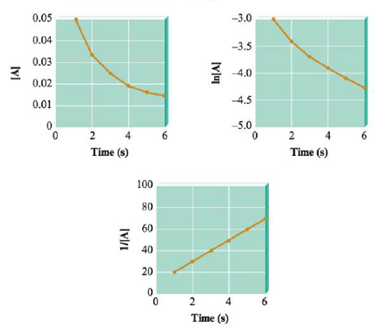# Experimental data for the reaction A → 2 B + C have been plotted in the following three different ways (with concentration units in mol/L): What is the order of the reaction with respect to A, and what is the initial concentration of A?### Chemistry: An Atoms First Approach

2nd Edition
Steven S. Zumdahl + 1 other
Publisher: Cengage Learning
ISBN: 9781305079243

#### Solutions

Chapter
Section### Chemistry: An Atoms First Approach

2nd Edition
Steven S. Zumdahl + 1 other
Publisher: Cengage Learning
ISBN: 9781305079243
Chapter 11, Problem 45E
Textbook Problem
45 views

## Experimental data for the reaction A → 2 B + C have been plotted in the following three different ways (with concentration units in mol/L):What is the order of the reaction with respect to A, and what is the initial concentration of A?

Interpretation Introduction

Interpretation: The general reaction is given. The graph of [A] versus time, ln[A] versus time and 1/[A] versus time is given. The order of reaction with respect to A and its initial concentration is to be calculated.

Concept introduction: The change observed in the concentration of a reactant or a product per unit time is known as the rate of the particular reaction. The differential rate

law provides the rate of a reaction at specific reaction concentrations.

To determine: The order of reaction with respect to A .

### Explanation of Solution

The given reaction is,

A2B+C

The rate law gives the relation between reaction rate and concentration of reactants. The rate law is represented as,

Rate=k[A]a (1)

Where,

• k is rate constant.
• [A] is concentration of reactant.
• a is reaction order of A.

The order of the given reaction can be determined by using following parameters,

• For first order reaction, ln[A]vstime graph is straight line
• For second order reaction, 1[A]vstime graph is straight line.
• For zero order reaction, [A]vstime graph is straight line.

Theplot of 1[A]vstime graphis straight line. It means that the given reaction is second order (a=2) reaction.

To determine: The initial concentration of A .

The integral rate law equation of second order reaction is,

1[A]=kt+1[A]0 (2)

Where,

• k is rate constant.
• [A]0 is initial concentration.
• [A] is final concentration.
• t is time.

The graph of second order reaction is,

Figure 1

Data from graph,

At t=6 the value of concentration is [A]=70

### Still sussing out bartleby?

Check out a sample textbook solution.

See a sample solution

#### The Solution to Your Study Problems

Bartleby provides explanations to thousands of textbook problems written by our experts, many with advanced degrees!

Get Started

Find more solutions based on key concepts
The eating pattern that best provides nutrients- singles out a rich source for each nutrient and focuses on the...

Nutrition: Concepts and Controversies - Standalone book (MindTap Course List)

Describe what happens during transformation.

General, Organic, and Biological Chemistry

What are the three most common chronic conditions affecting men over age 65?

Nutrition Through the Life Cycle (MindTap Course List)

When high-energy charged particles move through a transparent medium with a speed greater than the speed of lig...

Physics for Scientists and Engineers, Technology Update (No access codes included)